Courses

# Test: Design Of Bearings (Level - 1)

## 15 Questions MCQ Test Machine Design | Test: Design Of Bearings (Level - 1)

Description
This mock test of Test: Design Of Bearings (Level - 1) for Mechanical Engineering helps you for every Mechanical Engineering entrance exam. This contains 15 Multiple Choice Questions for Mechanical Engineering Test: Design Of Bearings (Level - 1) (mcq) to study with solutions a complete question bank. The solved questions answers in this Test: Design Of Bearings (Level - 1) quiz give you a good mix of easy questions and tough questions. Mechanical Engineering students definitely take this Test: Design Of Bearings (Level - 1) exercise for a better result in the exam. You can find other Test: Design Of Bearings (Level - 1) extra questions, long questions & short questions for Mechanical Engineering on EduRev as well by searching above.
QUESTION: 1

Solution:
QUESTION: 2

### If the load on a ball bearing is reduced to half, the life of the ball bearing will be B

Solution:

Given, Initially, w1 = w

Life = L1

After half of load, w2 =w/2

Life = L2

Considering the relation,

Lw3 = c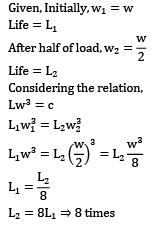L2 = 8L1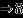times

QUESTION: 3

### Which of the following is not the part of roller bearing?

Solution:
QUESTION: 4

The life of bearing is expressed in

Solution:

Life of bearings is generally expressed as millions of revolution.

QUESTION: 5

Spherical roller bearings are normally used

Solution:
QUESTION: 6

Antifriction bearings are termed as

Solution:
QUESTION: 7

The rolling element bearings are

Solution:
QUESTION: 8

In a journal bearing P = average bearing pressure, Z = absolute viscosity of the lubricant, N = rotational speed of the journal. The bearing characteristic number is given by

Solution:
QUESTION: 9

With a dynamic load capacity of 2.2 kN, a roller bearing can operate at 600 rpm for 2000 hours. Then its maximum radial load will be equal to

Solution:

Given: Dynamic load capacity (C) = 2.2 kN

Life of bearing (Lf) = 60 x N x time duration

= 60 x 600 x 2000

= 72 x 106 revolutions

Using the following relation,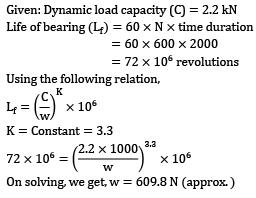K = Constant = 3.3On solving, we get, w = 609.8 (approx)

QUESTION: 10

A sliding bearing that can support steady loads without any relative motion between the journal and the bearing is called as

Solution:
QUESTION: 11

The expected life of a ball bearing subjected to a load of 9800 N and working at 1000 rpm is 3000 hours. Then the expected life of the same bearing for a similar load of 4900 N and speed of 2000 rpm will be

Solution:

Given: Load(w1 9800 N

Speed(N1) = 100 rpm

(Life) (L1)time duration = 3000 hours

Now, if load (w2) = 4900 N

Speed(N2) = 2000rpm

Then life(L2) = ？

Considering the following,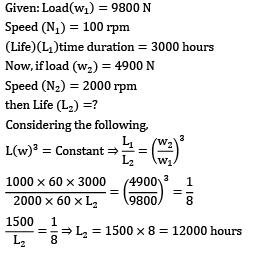QUESTION: 12

The dynamic load capacity of 6306 bearing is 22 kN. The maximum radial load it can sustain to operate at 600 rev/min, for 2000 hours will be equal to

Solution:

Given:dynamic load capacity = 22 x 103 N

Speed(N) = 600 rav/min

Life = 2000 x 60 x 600

72 x 106 rev

Using following relation,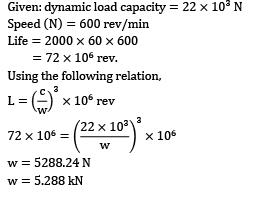w = 5288.24 N

w = 5.288 kN

QUESTION: 13

Which of the following assumptions regarding the lubricant film is made in Petroff’s equation?

Solution:
QUESTION: 14

In hydrodynamic bearings

Solution:
QUESTION: 15

The rated life of a bearing varies

Solution: